## Benford’s law emerges from deep language model

I was experimenting with the digits distribution from a pre-trained (weights from the OpenAI repositoryTransformer language model (LM) and I found a very interesting correlation between the Benford’s law and the digit distribution of the language model after conditioning it with some particular phrases.

Below is the correlation between the Benford’s law and the language model with conditioning on the phrase (shown in the figure):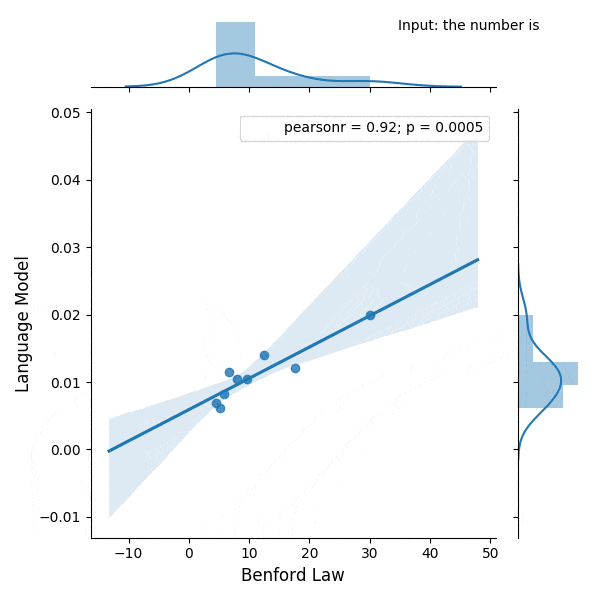## PyTorch 1.0 tracing JIT and LibTorch C++ API to integrate PyTorch into NodeJSUpdate 28 Feb 2019: I added a new blog post with a slide deck containing the presentation I did for PyData Montreal.

Today, at the PyTorch Developer Conference, the PyTorch team announced the plans and the release of the PyTorch 1.0 preview with many nice features such as a JIT for model graphs (with and without tracing) as well as the LibTorch, the PyTorch C++ API, one of the most important release announcement made today in my opinion.

Given the huge interest in understanding how this new API works, I decided to write this article showing an example of many opportunities that are now open after the release of the PyTorch C++ API. In this post, I’ll integrate PyTorch inference into native NodeJS using NodeJS C++ add-ons, just as an example of integration between different frameworks/languages that are now possible using the C++ API.

Below you can see the final result: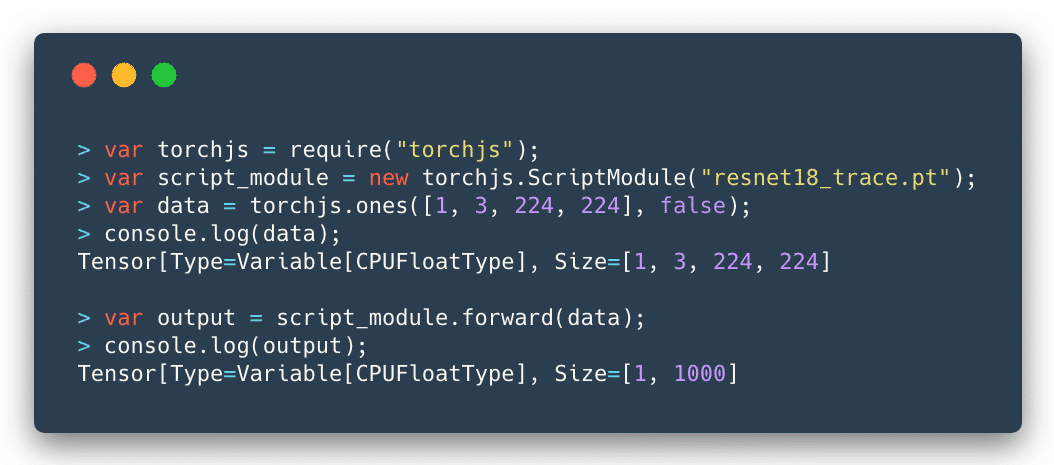As you can see, the integration is seamless and I could use a traced ResNet as the computational graph model and feed any tensor to it to get the output predictions.

### Introduction

Simply put, the libtorch is a library version of the PyTorch. It contains the underlying foundation that is used by PyTorch, such as the ATen (the tensor library), which contains all the tensor operations and methods. Libtorch also contains the autograd, which is the component that adds the automatic differentiation to the ATen tensors.

A word of caution for those who are starting now is to be careful with the use of the tensors that can be created both from ATen and autograd, do not mix them, the ATen will return the plain tensors (when you create them using the at namespace) while the autograd functions (from the torch namespace) will return Variable, by adding its automatic differentiation mechanism.

For a more extensive tutorial on how PyTorch internals work, please take a look on my previous tutorial on the PyTorch internal architecture.

Libtorch can be downloaded from the Pytorch website and it is only available as a preview for a while. You can also find the documentation in this site, which is mostly a Doxygen rendered documentation. I found the library pretty stable, and it makes sense because it is actually exposing the stable foundations of PyTorch, however, there are some issues with headers and some minor problems concerning the library organization that you might find while starting working with it (that will hopefully be fixed soon).For NodeJS, I’ll use the Native Abstractions library (nan) which is the most recommended library (actually is basically a header-only library) to create NodeJS C++ add-ons and the cmake-js, because libtorch already provide the cmake files that make our building process much easier. However, the focus here will be on the C++ code and not on the building process.The flow for the development, tracing, serializing and loading the model can be seen in the figure on the left side.

It starts with the development process and tracing being done in PyTorch (Python domain) and then the loading and inference on the C++ domain (in our case in NodeJS add-on).

### Wrapping the Tensor

In NodeJS, to create an object as a first-class citizen of the JavaScript world, you need to inherit from the ObjectWrap class, which will be responsible for wrapping a C++ component.

#ifndef TENSOR_H
#define TENSOR_H

#include <nan.h>
#include <torch/torch.h>

namespace torchjs {

class Tensor : public Nan::ObjectWrap {
public:
static NAN_MODULE_INIT(Init);

void setTensor(at::Tensor tensor) {
this->mTensor = tensor;
}

torch::Tensor getTensor() {
return this->mTensor;
}

static v8::Local<v8::Object> NewInstance();

private:
explicit Tensor();
~Tensor();

static NAN_METHOD(New);
static NAN_METHOD(toString);
static Nan::Persistent<v8::Function> constructor;

private:
torch::Tensor mTensor;

};

}  // namespace torchjs

#endif

As you can see, most of the code for the definition of our Tensor class is just boilerplate. The key point here is that the torchjs::Tensor will wrap a torch::Tensor and we added two special public methods (setTensor and getTensor) to set and get this internal torch tensor.I won’t show all the implementation details because most parts of it are NodeJS boilerplate code to construct the object, etc. I’ll focus on the parts that touch the libtorch API, like in the code below where we are creating a small textual representation of the tensor to show on JavaScript (toString method):

NAN_METHOD(Tensor::toString) {
Tensor* obj = ObjectWrap::Unwrap<Tensor>(info.Holder());
std::stringstream ss;

at::IntList sizes = obj->mTensor.sizes();
ss << "Tensor[Type=" << obj->mTensor.type() << ", ";
ss << "Size=" << sizes << std::endl;

info.GetReturnValue().Set(Nan::New(ss.str()).ToLocalChecked());
}

What we are doing in the code above, is just getting the internal tensor object from the wrapped object by unwrapping it. After that, we build a string representation with the tensor size (each dimension sizes) and its type (float, etc).

### Wrapping Tensor-creation operations

Let’s create now a wrapper code for the torch::ones function which is responsible for creating a tensor of any defined shape filled with constant 1’s.

NAN_METHOD(ones) {
// Sanity checking of the arguments
if (info.Length() < 2)
return Nan::ThrowError(Nan::New("Wrong number of arguments").ToLocalChecked());

if (!info->IsArray() || !info->IsBoolean())
return Nan::ThrowError(Nan::New("Wrong argument types").ToLocalChecked());

// Retrieving parameters (require_grad and tensor shape)
const v8::Local<v8::Array> array = info.As<v8::Array>();
const uint32_t length = array->Length();

// Convert from v8::Array to std::vector
std::vector<long long> dims;
for(int i=0; i<length; i++)
{
v8::Local<v8::Value> v;
int d = array->Get(i)->NumberValue();
dims.push_back(d);
}

// Call the libtorch and create a new torchjs::Tensor object
// wrapping the new torch::Tensor that was created by torch::ones
auto newinst = Tensor::NewInstance();
Tensor* obj = Nan::ObjectWrap::Unwrap<Tensor>(newinst);
obj->setTensor(v);

info.GetReturnValue().Set(newinst);
}

So, let’s go through this code. We are first checking the arguments of the function. For this function, we’re expecting a tuple (a JavaScript array) for the tensor shape and a boolean indicating if we want to compute gradients or not for this tensor node. After that, we’re converting the parameters from the V8 JavaScript types into native C++ types. Soon as we have the required parameters, we then call the torch::ones function from the libtorch, this function will create a new tensor where we use a torchjs::Tensor class that we created earlier to wrap it.

And that’s it, we just exposed one torch operation that can be used as native JavaScript operation.

### Intermezzo for the PyTorch JIT

The introduced PyTorch JIT revolves around the concept of the Torch Script. A Torch Script is a restricted subset of the Python language and comes with its own compiler and transform passes (optimizations, etc).

This script can be created in two different ways: by using a tracing JIT or by providing the script itself. In the tracing mode, your computational graph nodes will be visited and operations recorded to produce the final script, while the scripting is the mode where you provide this description of your model taking into account the restrictions of the Torch Script.

Note that if you have branching decisions on your code that depends on external factors or data, tracing won’t work as you expect because it will record that particular execution of the graph, hence the alternative option to provide the script. However, in most of the cases, the tracing is what we need.

To understand the differences, let’s take a look at the Intermediate Representation (IR) from the script module generated both by tracing and by scripting.

@torch.jit.script
def happy_function_script(x):
ret = torch.rand(0)
if True == True:
ret = torch.rand(1)
else:
ret = torch.rand(2)
return ret

def happy_function_trace(x):
ret = torch.rand(0)
if True == True:
ret = torch.rand(1)
else:
ret = torch.rand(2)
return ret

traced_fn = torch.jit.trace(happy_function_trace,
(torch.tensor(0),),
check_trace=False)

In the code above, we’re providing two functions, one is using the @torch.jit.script decorator, and it is the scripting way to create a Torch Script, while the second function is being used by the tracing function torch.jit.trace. Not that I intentionally added a “True == True” decision on the functions (which will always be true).

Now, if we inspect the IR generated by these two different approaches, we’ll clearly see the difference between the tracing and scripting approaches:

# 1) Graph from the scripting approach
graph(%x : Dynamic) {
%16 : int = prim::Constant[value=2]()
%10 : int = prim::Constant[value=1]()
%7 : int = prim::Constant[value=1]()
%8 : int = prim::Constant[value=1]()
%9 : int = aten::eq(%7, %8)
%ret : Dynamic = prim::If(%9)
block0() {
%11 : int[] = prim::ListConstruct(%10)
%12 : int = prim::Constant[value=6]()
%13 : int = prim::Constant[value=0]()
%14 : int[] = prim::Constant[value=[0, -1]]()
%ret.2 : Dynamic = aten::rand(%11, %12, %13, %14)
-> (%ret.2)
}
block1() {
%17 : int[] = prim::ListConstruct(%16)
%18 : int = prim::Constant[value=6]()
%19 : int = prim::Constant[value=0]()
%20 : int[] = prim::Constant[value=[0, -1]]()
%ret.3 : Dynamic = aten::rand(%17, %18, %19, %20)
-> (%ret.3)
}
return (%ret);
}

# 2) Graph from the tracing approach
graph(%0 : Long()) {
%7 : int = prim::Constant[value=1]()
%8 : int[] = prim::ListConstruct(%7)
%9 : int = prim::Constant[value=6]()
%10 : int = prim::Constant[value=0]()
%11 : int[] = prim::Constant[value=[0, -1]]()
%12 : Float(1) = aten::rand(%8, %9, %10, %11)
return (%12);
}

​

As we can see, the IR is very similar to the LLVM IR, note that in the tracing approach, the trace recorded contains only one path from the code, the truth path, while in the scripting we have both branching alternatives. However, even in scripting, the always false branch can be optimized and removed with a dead code elimination transform pass.

PyTorch JIT has a lot of transformation passes that are used to do loop unrolling, dead code elimination, etc. You can find these passes here. Not that conversion to other formats such as ONNX can be implemented as a pass on top of this intermediate representation (IR), which is quite convenient.

### Tracing the ResNet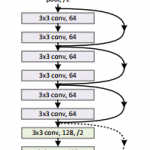Now, before implementing the Script Module in NodeJS, let’s first trace a ResNet network using PyTorch (using just Python):

traced_net = torch.jit.trace(torchvision.models.resnet18(),
torch.rand(1, 3, 224, 224))
traced_net.save("resnet18_trace.pt")

As you can see from the code above, we just have to provide a tensor example (in this case a batch of a single image with 3 channels and size 224×224. After that we just save the traced network into a file called resnet18_trace.pt.

Now we’re ready to implement the Script Module in NodeJS in order to load this file that was traced.

### Wrapping the Script Module

This is now the implementation of the Script Module in NodeJS:

// Class constructor
ScriptModule::ScriptModule(const std::string filename) {
// Load the traced network from the file
}

// JavaScript object creation
NAN_METHOD(ScriptModule::New) {
if (info.IsConstructCall()) {
// Get the filename parameter
v8::String::Utf8Value param_filename(info->ToString());
const std::string filename = std::string(*param_filename);

// Create a new script module using that file name
ScriptModule *obj = new ScriptModule(filename);
obj->Wrap(info.This());
info.GetReturnValue().Set(info.This());
} else {
v8::Local<v8::Function> cons = Nan::New(constructor);
info.GetReturnValue().Set(Nan::NewInstance(cons).ToLocalChecked());
}
}


As you can see from the code above, we’re just creating a class that will call the torch::jit::load function passing a file name of the traced network. We also have the implementation of the JavaScript object, where we convert parameters to C++ types and then create a new instance of the torchjs::ScriptModule.

The wrapping of the forward pass is also quite straightforward:

NAN_METHOD(ScriptModule::forward) {
ScriptModule* script_module = ObjectWrap::Unwrap<ScriptModule>(info.Holder());
Nan::MaybeLocal<v8::Object> maybe = Nan::To<v8::Object>(info);

Tensor *tensor =
Nan::ObjectWrap::Unwrap<Tensor>(maybe.ToLocalChecked());

torch::Tensor torch_tensor = tensor->getTensor();
torch::Tensor output = script_module->mModule->forward({torch_tensor}).toTensor();

auto newinst = Tensor::NewInstance();
Tensor* obj = Nan::ObjectWrap::Unwrap<Tensor>(newinst);
obj->setTensor(output);
info.GetReturnValue().Set(newinst);
}

As you can see, in this code, we just receive a tensor as an argument, we get the internal torch::Tensor from it and then call the forward method from the script module, we wrap the output on a new torchjs::Tensor and then return it.

And that’s it, we’re ready to use our built module in native NodeJS as in the example below:

var torchjs = require("./build/Release/torchjs");
var script_module = new torchjs.ScriptModule("resnet18_trace.pt");
var data = torchjs.ones([1, 3, 224, 224], false);
var output = script_module.forward(data);

I hope you enjoyed ! Libtorch opens the door for the tight integration of PyTorch in many different languages and frameworks, which is quite exciting and a huge step towards the direction of production deployment code.

– Christian S. Perone

Cite this article as: Christian S. Perone, "PyTorch 1.0 tracing JIT and LibTorch C++ API to integrate PyTorch into NodeJS," in Terra Incognita, 02/10/2018, https://blog.christianperone.com/2018/10/pytorch-1-0-tracing-jit-and-libtorch-c-api-to-integrate-pytorch-into-nodejs/.

## PyTorch – Internal Architecture Tour

Update 28 Feb 2019: I added a new blog post with a slide deck containing the presentation I did for PyData Montreal.

## Introduction

This post is a tour around the PyTorch codebase, it is meant to be a guide for the architectural design of PyTorch and its internals. My main goal is to provide something useful for those who are interested in understanding what happens beyond the user-facing API and show something new beyond what was already covered in other tutorials.

Note: PyTorch build system uses code generation extensively so I won’t repeat here what was already described by others. If you’re interested in understanding how this works, please read the following tutorials:

## Short intro to Python extension objects in C/C++

As you probably know, you can extend Python using C and C++ and develop what is called as “extension”. All the PyTorch heavy work is implemented in C/C++ instead of pure-Python. To define a new Python object type in C/C++, you define a structure like this one example below (which is the base for the autograd Variable class):

// Python object that backs torch.autograd.Variable
struct THPVariable {
PyObject* backward_hooks;
};

As you can see, there is a macro at the beginning of the definition, called PyObject_HEAD, this macro’s goal is the standardization of Python objects and will expand to another structure that contains a pointer to a type object (which defines initialization methods, allocators, etc) and also a field with a reference counter.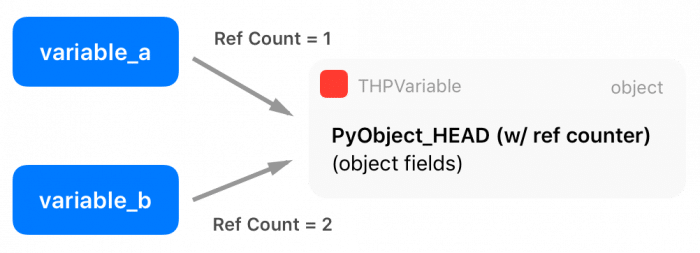There are two extra macros in the Python API called Py_INCREF() and Py_DECREF(), which are used to increment and decrement the reference counter of Python objects. Multiple entities can borrow or own a reference to other objects (the reference counter is increased), and only when this reference counter reaches zero (when all references get destroyed), Python will automatically delete the memory from that object using its garbage collector.

Funny fact: it is very common in many applications to use small integer numbers as indexing, counters, etc. For efficiency, the official CPython interpreter caches the integers from -5 up to 256. For that reason, the statement a = 200; b = 200; a is b will be True, while the statement a = 300; b = 300; a is b will be False.

## Zero-copy PyTorch Tensor to Numpy and vice-versa

PyTorch has its own Tensor representation, which decouples PyTorch internal representation from external representations. However, as it is very common, especially when data is loaded from a variety of sources, to have Numpy arrays everywhere, therefore we really need to make conversions between Numpy and PyTorch tensors. For that reason, PyTorch provides two methods called from_numpy() and numpy(), that converts a Numpy array to a PyTorch array and vice-versa, respectively. If we look the code that is being called to convert a Numpy array into a PyTorch tensor, we can get more insights on the PyTorch’s internal representation:

at::Tensor tensor_from_numpy(PyObject* obj) {
if (!PyArray_Check(obj)) {
throw TypeError("expected np.ndarray (got %s)", Py_TYPE(obj)->tp_name);
}

auto array = (PyArrayObject*)obj;
int ndim = PyArray_NDIM(array);
auto sizes = to_aten_shape(ndim, PyArray_DIMS(array));
auto strides = to_aten_shape(ndim, PyArray_STRIDES(array));
// NumPy strides use bytes. Torch strides use element counts.
auto element_size_in_bytes = PyArray_ITEMSIZE(array);
for (auto& stride : strides) {
stride /= element_size_in_bytes;
}

// (...) - omitted for brevity

void* data_ptr = PyArray_DATA(array);
auto& type = CPU(dtype_to_aten(PyArray_TYPE(array)));
Py_INCREF(obj);
return type.tensorFromBlob(data_ptr, sizes, strides, [obj](void* data) {
AutoGIL gil;
Py_DECREF(obj);
});
}

(code from tensor_numpy.cpp)

As you can see from this code, PyTorch is obtaining all information (array metadata) from Numpy representation and then creating its own. However, as you can note from the marked line 18, PyTorch is getting a pointer to the internal Numpy array raw data instead of copying it. This means that PyTorch will create a reference for this data, sharing the same memory region with the Numpy array object for the raw Tensor data.There is also an important point here: when Numpy array object goes out of scope and get a zero reference count, it will be garbage collected and destroyed, that’s why there is an increment in the reference counting of the Numpy array object at line 20.

After this, PyTorch will create a new Tensor object from this Numpy data blob, and in the creation of this new Tensor it passes the borrowed memory data pointer, together with the memory size and strides as well as a function that will be used later by the Tensor Storage (we’ll discuss this in the next section) to release the data by decrementing the reference counting to the Numpy array object and let Python take care of this object life cycle.

The tensorFromBlob() method will create a new Tensor, but only after creating a new “Storage” for this Tensor. The storage is where the actual data pointer will be stored (and not in the Tensor structure itself). This takes us to the next section about Tensor Storages.

## Tensor Storage

The actual raw data of the Tensor is not directly kept in the Tensor structure, but on another structure called Storage, which in turn is part of the Tensor structure.

As we saw in the previous code from tensor_from_numpy(), there is a call for tensorFromBlob() that will create a Tensor from the raw data blob. This last function will call another function called storageFromBlob() that will, in turn, create a storage for this data according to its type. In the case of a CPU float type, it will return a new CPUFloatStorage instance.

The CPUFloatStorage is basically a wrapper with utility functions around the actual storage structure called THFloatStorage that we show below:

typedef struct THStorage
{
real *data;
ptrdiff_t size;
int refcount;
char flag;
THAllocator *allocator;
void *allocatorContext;
struct THStorage *view;
} THStorage;

(code from THStorage.h)

As you can see, the THStorage holds a pointer to the raw data, its size, flags and also an interesting field called allocator that we’ll soon discuss. It is also important to note that there is no metadata regarding on how to interpret the data inside the THStorage, this is due to the fact that the storage is “dumb” regarding of its contents and it is the Tensor responsibility to know how to “view” or interpret this data.

From this, you already probably realized that we can have multiple tensors pointing to the same storage but with different views of this data, and that’s why viewing a tensor with a different shape (but keeping the same number of elements) is so efficient. This Python code below shows that the data pointer in the storage is being shared after changing the way Tensor views its data:

>>> tensor_a = torch.ones((3, 3))
>>> tensor_b = tensor_a.view(9)
>>> tensor_a.storage().data_ptr() == tensor_b.storage().data_ptr()
True

As we can see in the example above, the data pointer on the storage of both Tensors are the same, but the Tensors represent a different interpretation of the storage data.

Now, as we saw in line 7 of the THFloatStorage structure, there is a pointer to a THAllocator structure there. And this is very important because it brings flexibility regarding the allocator that can be used to allocate the storage data. This structure is represented by the following code:

typedef struct THAllocator
{
void* (*malloc)(void*, ptrdiff_t);
void* (*realloc)(void*, void*, ptrdiff_t);
void (*free)(void*, void*);
} THAllocator;

(code from THAllocator.h)

As you can see, there are three function pointer fields in this structure to define what an allocator means: a malloc, realloc and free. For CPU-allocated memory, these functions will, of course, relate to the traditional malloc/realloc/free POSIX functions, however, when we want a storage allocated on GPUs we’ll end up using the CUDA allocators such as the cudaMallocHost(), like we can see in the THCudaHostAllocator malloc function below:

static void *THCudaHostAllocator_malloc(void* ctx, ptrdiff_t size) {
void* ptr;
if (size < 0) THError("Invalid memory size: %ld", size);
if (size == 0) return NULL;
THCudaCheck(cudaMallocHost(&ptr, size));
return ptr;
}

(code from THCAllocator.c)

You probably noticed a pattern in the repository organization, but it is important to keep in mind these conventions when navigating the repository, as summarized here (taken from the PyTorch lib readme):

• TH = TorcH
• THC = TorcH Cuda
• THCS = TorcH Cuda Sparse
• THCUNN = TorcH CUda Neural Network
• THD = TorcH Distributed
• THNN = TorcH Neural Network
• THS = TorcH Sparse

This convention is also present in the function/class names and other objects, so it is important to always keep these patterns in mind. While you can find CPU allocators in the TH code, you’ll find CUDA allocators in the THC code.

Finally, we can see the composition of the main Tensor THTensor structure:

typedef struct THTensor
{
int64_t *size;
int64_t *stride;
int nDimension;
THStorage *storage;
ptrdiff_t storageOffset;
int refcount;
char flag;
} THTensor;

(Code from THTensor.h)

And as you can see, the main THTensor structure holds the size/strides/dimensions/offsets/etc as well as the storage (THStorage) for the Tensor data.

We can summarize all this structure that we saw in the diagram below: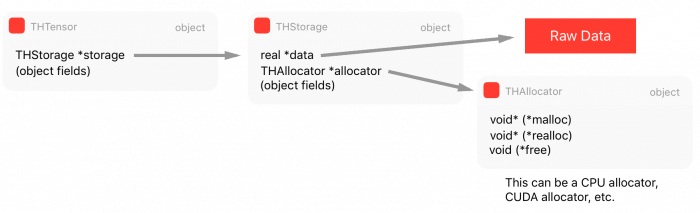Now, once we have requirements such as multi-processing where we want to share tensor data among multiple different processes, we need a shared memory approach to solve it, otherwise, every time another process needs a tensor or even when you want to implement Hogwild training procedure where all different processes will write to the same memory region (where the parameters are), you’ll need to make copies between processes, and this is very inefficient. Therefore we’ll discuss in the next section a special kind of storage for Shared Memory.

## Shared Memory

Shared memory can be implemented in many different ways depending on the platform support. PyTorch supports some of them, but for the sake of simplicity, I’ll talk here about what happens on MacOS using the CPU (instead of GPU). Since PyTorch supports multiple shared memory approaches, this part is a little tricky to grasp into since it involves more levels of indirection in the code.

PyTorch provides a wrapper around the Python multiprocessing module and can be imported from torch.multiprocessing. The changes they implemented in this wrapper around the official Python multiprocessing were done to make sure that everytime a tensor is put on a queue or shared with another process, PyTorch will make sure that only a handle for the shared memory will be shared instead of a new entire copy of the Tensor.

Now, many people aren’t aware of a Tensor method from PyTorch called share_memory_(), however, this function is what triggers an entire rebuild of the storage memory for that particular Tensor. What this method does is to create a region of shared memory that can be used among different processes. This function will, in the end, call this following function below:

static THStorage* THPStorage_(newFilenameStorage)(ptrdiff_t size)
{
int flags = TH_ALLOCATOR_MAPPED_SHAREDMEM | TH_ALLOCATOR_MAPPED_EXCLUSIVE;
std::string handle = THPStorage_(__newHandle)();
auto ctx = libshm_context_new(NULL, handle.c_str(), flags);
return THStorage_(newWithAllocator)(size, &THManagedSharedAllocator, (void*)ctx);
}

(Code from StorageSharing.cpp)

And as you can see, this function will create another storage using a special allocator called THManagedSharedAllocator. This function first defines some flags and then it creates a handle which is a string in the format /torch_[process id]_[random number], and after that, it will then create a new storage using the special THManagedSharedAllocator. This allocator has function pointers to an internal PyTorch library called libshm, that will implement a Unix Domain Socket communication to share the shared memory region handles. This allocator is actual an especial case and it is a kind of “smart allocator” because it contains the communication control logic as well as it uses another allocator called THRefcountedMapAllocator that will be responsible for creating the actual shared memory region and call mmap() to map this region to the process virtual address space.

Note: when a method ends with a underscore in PyTorch, such as the method called share_memory_(), it means that this method has an in-place effect, and it will change the current object instead of creating a new one with the modifications.

I’ll now show a Python example of one processing using the data from a Tensor that was allocated on another process by manually exchanging the shared memory handle:

This is executed in the process A:

>>> import torch
>>> tensor_a = torch.ones((5, 5))
>>> tensor_a

1  1  1  1  1
1  1  1  1  1
1  1  1  1  1
1  1  1  1  1
1  1  1  1  1
[torch.FloatTensor of size 5x5]

>>> tensor_a.is_shared()
False
>>> tensor_a = tensor_a.share_memory_()
>>> tensor_a.is_shared()
True
>>> tensor_a_storage = tensor_a.storage()
>>> tensor_a_storage._share_filename_()
(b'/var/tmp/tmp.0.yowqlr', b'/torch_31258_1218748506', 25)

In this code, executed in the process A, we create a new Tensor of 5×5 filled with ones. After that we make it shared and print the tuple with the Unix Domain Socket address as well as the handle. Now we can access this memory region from another process B as shown below:

Code executed in the process B:

>>> import torch
>>> tensor_a = torch.Tensor()
>>> tuple_info = (b'/var/tmp/tmp.0.yowqlr', b'/torch_31258_1218748506', 25)
>>> storage = torch.Storage._new_shared_filename(*tuple_info)
>>> tensor_a = torch.Tensor(storage).view((5, 5))

1  1  1  1  1
1  1  1  1  1
1  1  1  1  1
1  1  1  1  1
1  1  1  1  1
[torch.FloatTensor of size 5x5]

As you can see, using the tuple information about the Unix Domain Socket address and the handle we were able to access the Tensor storage from another process. If you change the tensor in this process B, you’ll also see that it will reflect in the process A because these Tensors are sharing the same memory region.

## DLPack: a hope for the Deep Learning frameworks Babel

Now I would like to talk about something recent in the PyTorch code base, that is called DLPack. DLPack is an open standardization of an in-memory tensor structure that will allow exchange tensor data between frameworks, and what is quite interesting is that since this memory representation is standardized and very similar to the memory representation already in use by many frameworks, it will allow a zero-copy data sharing between frameworks, which is a quite amazing initiative given the variety of frameworks we have today without inter-communication among them.

This will certainly help to overcome the “island model” that we have today between tensor representations in MXNet, PyTorch, etc, and will allow developers to mix framework operations between frameworks and all the benefits that a standardization can bring to the frameworks.

The core of DLPack os a very simple structure called DLTensor, as shown below:

/*!
* \brief Plain C Tensor object, does not manage memory.
*/
typedef struct {
/*!
* \brief The opaque data pointer points to the allocated data.
*  This will be CUDA device pointer or cl_mem handle in OpenCL.
*  This pointer is always aligns to 256 bytes as in CUDA.
*/
void* data;
/*! \brief The device context of the tensor */
DLContext ctx;
/*! \brief Number of dimensions */
int ndim;
/*! \brief The data type of the pointer*/
DLDataType dtype;
/*! \brief The shape of the tensor */
int64_t* shape;
/*!
* \brief strides of the tensor,
*  can be NULL, indicating tensor is compact.
*/
int64_t* strides;
/*! \brief The offset in bytes to the beginning pointer to data */
uint64_t byte_offset;
} DLTensor;

(code from dlpack.h)

As you can see, there is a data pointer for the raw data as well as shape/stride/offset/GPU vs CPU, and other metadata information about the data that the DLTensor pointing to.

There is also a managed version of the tensor that is called DLManagedTensor, where the frameworks can provide a context and also a “deleter” function that can be called by the framework who borrowed the Tensor to inform the other framework that the resources are no longer required.

In PyTorch, if you want to convert to or from a DLTensor format, you can find both C/C++ methods for doing that or even in Python you can do that as shown below:

import torch
from torch.utils import dlpack

t = torch.ones((5, 5))
dl = dlpack.to_dlpack(t)

This Python function will call the toDLPack function from ATen, shown below:

DLManagedTensor* toDLPack(const Tensor& src) {
ATenDLMTensor * atDLMTensor(new ATenDLMTensor);
atDLMTensor->handle = src;
atDLMTensor->tensor.manager_ctx = atDLMTensor;
atDLMTensor->tensor.deleter = &deleter;
atDLMTensor->tensor.dl_tensor.data = src.data_ptr();
int64_t device_id = 0;
if (src.type().is_cuda()) {
device_id = src.get_device();
}
atDLMTensor->tensor.dl_tensor.ctx = getDLContext(src.type(), device_id);
atDLMTensor->tensor.dl_tensor.ndim = src.dim();
atDLMTensor->tensor.dl_tensor.dtype = getDLDataType(src.type());
atDLMTensor->tensor.dl_tensor.shape = const_cast<int64_t*>(src.sizes().data());
atDLMTensor->tensor.dl_tensor.strides = const_cast<int64_t*>(src.strides().data());
atDLMTensor->tensor.dl_tensor.byte_offset = 0;
return &(atDLMTensor->tensor);
}

As you can see, it’s a pretty simple conversion, casting the metadata from the PyTorch format to the DLPack format and assigning a pointer to the internal Tensor data representation.

I really hope that more frameworks adopt this standard that will certainly give benefits to the ecosystem. It is also interesting to note that a potential integration with Apache Arrow would be amazing.

That’s it, I hope you liked this long post !

– Christian S. Perone

Cite this article as: Christian S. Perone, "PyTorch – Internal Architecture Tour," in Terra Incognita, 12/03/2018, https://blog.christianperone.com/2018/03/pytorch-internal-architecture-tour/.

## Privacy-preserving sentence semantic similarity using InferSent embeddings and secure two-party computation

### Privacy-preserving Computation

Privacy-preserving computation or secure computation is a sub-field of cryptography where two (two-party, or 2PC) or multiple (multi-party, or MPC) parties can evaluate a function together without revealing information about the parties private input data to each other. The problem and the first solution to it were introduced in 1982 by an amazing breakthrough done by Andrew Yao on what later became known as the “Yao’s Millionaires’ problem“.

The Yao’s Millionaires Problem is where two millionaires, Alice and Bob, who are interested in knowing which of them is richer but without revealing to each other their actual wealth. In other words, what they want can be generalized as that: Alice and Bob want jointly compute a function securely, without knowing anything other than the result of the computation on the input data (that remains private to them).

To make the problem concrete, Alice has an amount A such as $10, and Bob has an amount B such as$ 50, and what they want to know is which one is larger, without Bob revealing the amount B to Alice or Alice revealing the amount A to Bob. It is also important to note that we also don’t want to trust on a third-party, otherwise the problem would just be a simple protocol of information exchange with the trusted party.Formally what we want is to jointly evaluate the following function:$r = f(A, B)$

Such as the private values A and B are held private to the sole owner of it and where the result r will be known to just one or both of the parties.

It seems very counterintuitive that a problem like that could ever be solved, but for the surprise of many people, it is possible to solve it on some security requirements. Thanks to the recent developments in techniques such as FHE (Fully Homomorphic Encryption), Oblivious Transfer, Garbled Circuits, problems like that started to get practical for real-life usage and they are being nowadays being used by many companies in applications such as information exchange, secure location, advertisement, satellite orbit collision avoidance, etc.

I’m not going to enter into details of these techniques, but if you’re interested in the intuition behind the OT (Oblivious Transfer), you should definitely read the amazing explanation done by Craig Gidney here. There are also, of course, many different protocols for doing 2PC or MPC, where each one of them assumes some security requirements (semi-honest, malicious, etc), I’m not going to enter into the details to keep the post focused on the goal, but you should be aware of that.

### The problem: sentence similarity

What we want to achieve is to use privacy-preserving computation to calculate the similarity between sentences without disclosing the content of the sentences. Just to give a concrete example: Bob owns a company and has the description of many different projects in sentences such as: “This project is about building a deep learning sentiment analysis framework that will be used for tweets“, and Alice who owns another competitor company, has also different projects described in similar sentences.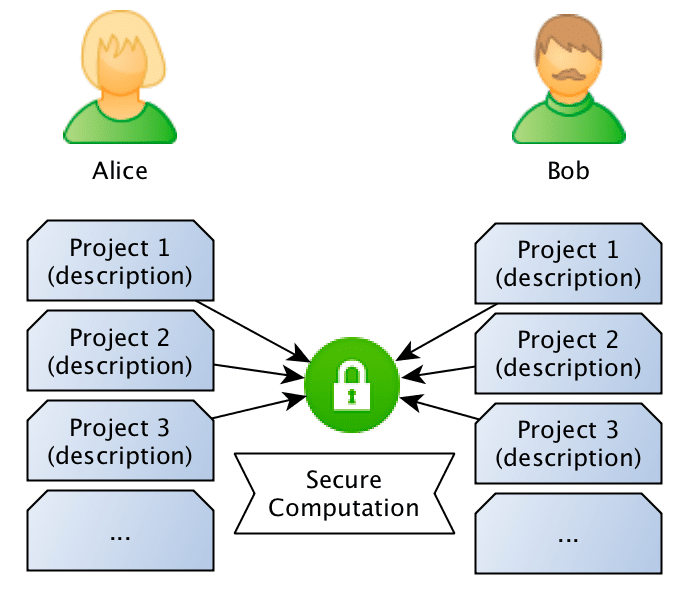What they want to do is to jointly compute the similarity between projects in order to find if they should be doing partnership on a project or not, however, and this is the important point: Bob doesn’t want Alice to know the project descriptions and neither Alice wants Bob to be aware of their projects, they want to know the closest match between the different projects they run, but without disclosing the project ideas (project descriptions).

### Sentence Similarity Comparison

Now, how can we exchange information about the Bob and Alice’s project sentences without disclosing information about the project descriptions ?

One naive way to do that would be to just compute the hashes of the sentences and then compare only the hashes to check if they match. However, this would assume that the descriptions are exactly the same, and besides that, if the entropy of the sentences is small (like small sentences), someone with reasonable computation power can try to recover the sentence.

Another approach for this problem (this is the approach that we’ll be using), is to compare the sentences in the sentence embeddings space. We just need to create sentence embeddings using a Machine Learning model (we’ll use InferSent later) and then compare the embeddings of the sentences. However, this approach also raises another concern: what if Bob or Alice trains a Seq2Seq model that would go from the embeddings of the other party back to an approximate description of the project ?

It isn’t unreasonable to think that one can recover an approximate description of the sentence given their embeddings. That’s why we’ll use the two-party secure computation for computing the embeddings similarity, in a way that Bob and Alice will compute the similarity of the embeddings without revealing their embeddings, keeping their project ideas safe.

The entire flow is described in the image below, where Bob and Alice shares the same Machine Learning model, after that they use this model to go from sentences to embeddings, followed by a secure computation of the similarity in the embedding space.

### Generating sentence embeddings with InferSent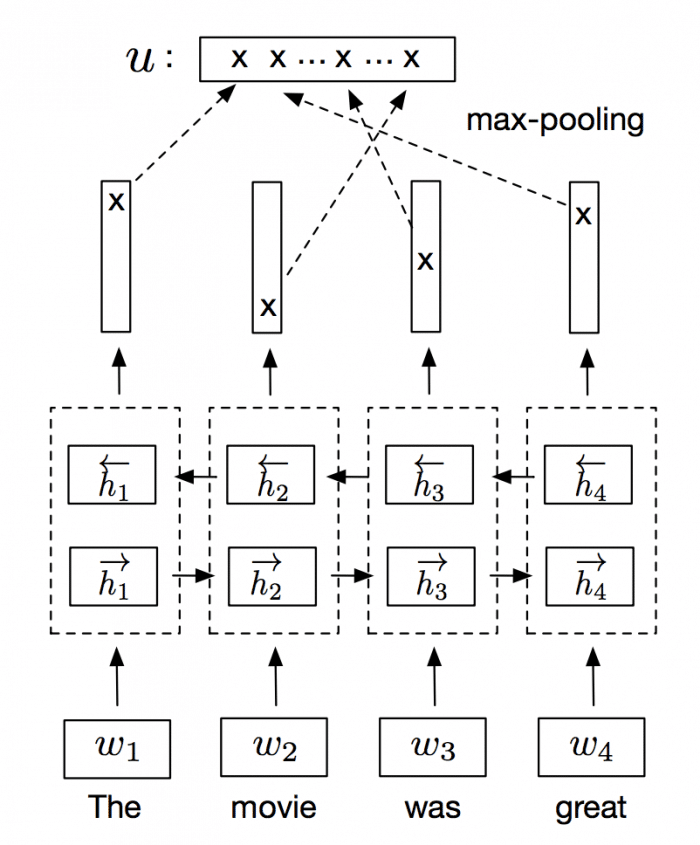Bi-LSTM max-pooling network. Source: Supervised Learning of Universal Sentence Representations from Natural Language Inference Data. Alexis Conneau et al.

InferSent is an NLP technique for universal sentence representation developed by Facebook that uses supervised training to produce high transferable representations.

They used a Bi-directional LSTM with attention that consistently surpassed many unsupervised training methods such as the SkipThought vectors. They also provide a Pytorch implementation that we’ll use to generate sentence embeddings.

Note: even if you don’t have GPU, you can have reasonable performance doing embeddings for a few sentences.

The first step to generate the sentence embeddings is to download and load a pre-trained InferSent model:

import numpy as np
import torch

GLOVE_EMBS = '../dataset/GloVe/glove.840B.300d.txt'
INFERSENT_MODEL = 'infersent.allnli.pickle'

map_location=lambda storage, loc: storage)

model.set_glove_path(GLOVE_EMBS)
model.build_vocab_k_words(K=100000)

Now we need to define a similarity measure to compare two vectors, and for that goal, I’ll the cosine similarity (I wrote a tutorial about this similarity measure here) since it’s pretty straightforward:$cos(\pmb x, \pmb y) = \frac {\pmb x \cdot \pmb y}{||\pmb x|| \cdot ||\pmb y||}$

As you can see, if we have two unit vectors (vectors with norm 1), the two terms in the equation denominator will be 1 and we will be able to remove the entire denominator of the equation, leaving only:$cos(\hat{x}, \hat{y}) =\hat{x} \cdot\hat{y}$

So, if we normalize our vectors to have a unit norm (that’s why the vectors are wearing hats in the equation above), we can make the computation of the cosine similarity become just a simple dot product. That will help us a lot in computing the similarity distance later when we’ll use a framework to do the secure computation of this dot product.

So, the next step is to define a function that will take some sentence text and forward it to the model to generate the embeddings and then normalize them to unit vectors:

# This function will forward the text into the model and
# get the embeddings. After that, it will normalize it
# to a unit vector.

def encode(model, text):
embedding = model.encode([text])
embedding /= np.linalg.norm(embedding)
return embedding

As you can see, this function is pretty simple, it feeds the text into the model, and then it will divide the embedding vector by the embedding norm.

Now, for practical reasons, I’ll be using integer computation later for computing the similarity, however, the embeddings generated by InferSent are of course real values. For that reason, you’ll see in the code below that we create another function to scale the float values and remove the radix point and converting them to integers. There is also another important issue, the framework that we’ll be using later for secure computation doesn’t allow signed integers, so we also need to clip the embeddings values between 0.0 and 1.0. This will of course cause some approximation errors, however, we can still get very good approximations after clipping and scaling with limited precision (I’m using 14 bits for scaling to avoid overflow issues later during dot product computations):

# This function will scale the embedding in order to

def scale(embedding):
SCALE = 1 << 14
scale_embedding = np.clip(embedding, 0.0, 1.0) * SCALE
return scale_embedding.astype(np.int32)


You can use floating-point in your secure computations and there are a lot of frameworks that support them, however, it is more tricky to do that, and for that reason, I used integer arithmetic to simplify the tutorial. The function above is just a hack to make it simple. It’s easy to see that we can recover this embedding later without too much loss of precision.

Now we just need to create some sentence samples that we’ll be using:

# The list of Alice sentences
alice_sentences = [
'my cat loves to walk over my keyboard',
'I like to pet my cat',
]

# The list of Bob sentences
bob_sentences = [
'the cat is always walking over my keyboard',
]

And convert them to embeddings:

# Alice sentences
alice_sentence1 = encode(model, alice_sentences)
alice_sentence2 = encode(model, alice_sentences)

# Bob sentences
bob_sentence1 = encode(model, bob_sentences)

Since we have now the sentences and every sentence is also normalized, we can compute cosine similarity just by doing a dot product between the vectors:

>>> np.dot(bob_sentence1, alice_sentence1)
0.8798542

>>> np.dot(bob_sentence1, alice_sentence2)
0.62976325

As we can see, the first sentence of Bob is most similar (~0.87) with Alice first sentence than to the Alice second sentence (~0.62).

Since we have now the embeddings, we just need to convert them to scaled integers:

# Scale the Alice sentence embeddings
alice_sentence1_scaled = scale(alice_sentence1)
alice_sentence2_scaled = scale(alice_sentence2)

# Scale the Bob sentence embeddings
bob_sentence1_scaled = scale(bob_sentence1)

# This is the unit vector embedding for the sentence
>>> alice_sentence1
array([ 0.01698913, -0.0014404 ,  0.0010993 , ...,  0.00252409,
0.00828147,  0.00466533], dtype=float32)

# This is the scaled vector as integers
>>> alice_sentence1_scaled
array([278,   0,  18, ...,  41, 135,  76], dtype=int32)

Now with these embeddings as scaled integers, we can proceed to the second part, where we’ll be doing the secure computation between two parties.

### Two-party secure computation

In order to perform secure computation between the two parties (Alice and Bob), we’ll use the ABY framework. ABY implements many difference secure computation schemes and allows you to describe your computation as a circuit like pictured in the image below, where the Yao’s Millionaire’s problem is described:

As you can see, we have two inputs entering in one GT GATE (greater than gate) and then a output. This circuit has a bit length of 3 for each input and will compute if the Alice input is greater than (GT GATE) the Bob input. The computing parties then secret share their private data and then can use arithmetic sharing, boolean sharing, or Yao sharing to securely evaluate these gates.

ABY is really easy to use because you can just describe your inputs, shares, gates and it will do the rest for you such as creating the socket communication channel, exchanging data when needed, etc. However, the implementation is entirely written in C++ and I’m not aware of any Python bindings for it (a great contribution opportunity).

Fortunately, there is an implemented example for ABY that can do dot product calculation for us, the example is here. I won’t replicate the example here, but the only part that we have to change is to read the embedding vectors that we created before instead of generating random vectors and increasing the bit length to 32-bits.

After that, we just need to execute the application on two different machines (or by emulating locally like below):

# This will execute the server part, the -r 0 specifies the role (server)
# and the -n 4096 defines the dimension of the vector (InferSent generates
# 4096-dimensional embeddings).
~# ./innerproduct -r 0 -n 4096

# And the same on another process (or another machine, however for another
# machine execution you'll have to obviously specify the IP).
~# ./innerproduct -r 1 -n 4096

And we get the following results:

Inner Product of alice_sentence1 and bob_sentence1  = 226691917
Inner Product of alice_sentence2 and bob_sentence1  = 171746521

Even in the integer representation, you can see that the inner product of the Alice’s first sentence and the Bob sentence is higher, meaning that the similarity is also higher. But let’s now convert this value back to float:

>>> SCALE = 1 << 14

# This is the dot product we should get
>>> np.dot(alice_sentence1, bob_sentence1)
0.8798542

# This is the inner product we got on secure computation
>>> 226691917 / SCALE**2.0
0.8444931

# This is the dot product we should get
>>> np.dot(alice_sentence2, bob_sentence1)
0.6297632

# This is the inner product we got on secure computation
>>> 171746521 / SCALE**2.0
0.6398056

As you can see, we got very good approximations, even in presence of low-precision math and unsigned integer requirements. Of course that in real-life you won’t have the two values and vectors, because they’re supposed to be hidden, but the changes to accommodate that are trivial, you just need to adjust ABY code to load only the vector of the party that it is executing it and using the correct IP addresses/port of the both parties.

I hope you liked it !

– Christian S. Perone

Cite this article as: Christian S. Perone, "Privacy-preserving sentence semantic similarity using InferSent embeddings and secure two-party computation," in Terra Incognita, 22/01/2018, https://blog.christianperone.com/2018/01/privacy-preserving-infersent/.

## The effective receptive field on CNNs

Given the interesting recent article on “The Emergence of a Fovea while Learning to Attend“, I decide to make a review of the paper written by Luo, Wenjie et al. called “Understanding the Effective Receptive Field in Deep Convolutional Neural Networks” where they introduced the idea of the “Effective Receptive Field” (ERF) and the surprising relationship with the foveal vision that arises naturally on Convolutional Neural Networks.

The receptive field in Convolutional Neural Networks (CNN) is the region of the input space that affects a particular unit of the network. Note that this input region can be not only the input of the network but also output from other units in the network, therefore this receptive field can be calculated relative to the input that we consider and also relative the unit that we are taking into consideration as the “receiver” of this input region. Usually, when the receptive field term is mentioned, it is taking into consideration the final output unit of the network (i.e. a single unit on a binary classification task) in relation to the network input (i.e. input image of the network).

It is easy to see that on a CNN, the receptive field can be increased using different methods such as: stacking more layers (depth), subsampling (pooling, striding), filter dilation (dilated convolutions), etc. In theory, when you stack more layers you can increase your receptive field linearly, however, in practice, things aren’t simple as we thought, as shown by Luo, Wenjie et al. article. In the article, they introduce the concept of the “Effective Receptive Field”, or ERF; the intuition behind the concept is that not all pixels in the receptive field contribute equally to the output unit’s response. When doing the forward pass, we can see that the central receptive field pixels can propagate their information to the output using many different paths, as they are part of multiple output unit’s calculations.

In the figure below, we can see in left the input pixels, after that we have a feature map calculated from the input pixels using a 3×3 convolution filter and then finally the output after another 3×3 filtering. The numbers inside the pixels on the left image represent how many times this pixel was part of a convolution step (each sliding step of the filter). As we can see, some pixels like the central ones will have their information propagated through many different paths in the network, while the pixels on the borders are propagated along a single path.

By looking at the image above, it isn’t that surprising that the effective receptive field impact on the final output computation will look more like a Gaussian distribution instead of a uniform distribution. What is actually more even interesting is that this receptive field is dynamic and changes during the training. The impact of this on the backpropagation is that the central pixels will have a larger gradient magnitude when compared to the border pixels.

In the article written by Luo, Wenjie et al., they devised a way to quantify the effect on each input pixel of the network by calculating the quantity$\frac{\partial y}{\partial x_{i, j}}$ that represents how much each pixel$x_{i, j}$ contributes to the output$y$.

In the paper, they did experimentations to visualize the effective receptive field using multiple different architectures, activations, etc. I replicate here the ones that I found most interesting: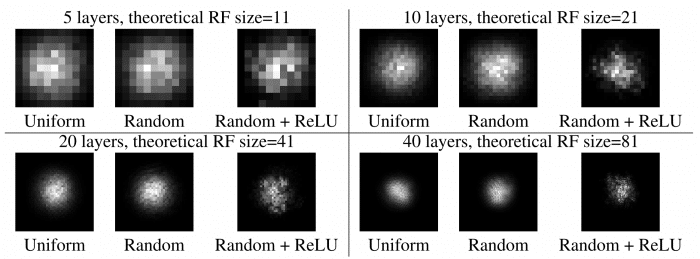Figure 1 from the paper “Understanding the Effective Receptive Field in Deep Convolutional Neural Networks”, by Luo, Wenjie et al.

As we can see from the Figure 1 of the paper, where they compare the effect of the number of layers, initialization schemes, and different activations, the results are amazing. We can clearly see the Gaussian and also the sparsity added by the ReLU activations.

There are also some comparisons on Figure 3 of the paper, where CIFAR-10 and CamVid datasets were used to train the network.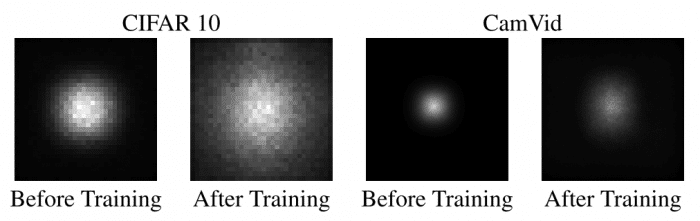Figure 3 of the paper “Understanding the Effective Receptive Field in Deep Convolutional Neural Networks”, by Luo, Wenjie et al.

As we can see, the size of the effective receptive field is very dynamic and it is increased by a large margin after the training, which implies, as stated by authors of the paper, that better initialization schemes can be employed to increase the receptive field in the beginning of the training. They actually developed a different initialization scheme and were able to get 30% training speed-up, however, these results weren’t consistent.

What is also very interesting, is that the effective receptive field has a very close relationship with the foveal vision of the human eye, which produces the sharp central vision, effect of the high-density region of cone cells (as shown in the image below) present in the eye fundus.

Our central sharp vision also decays rapidly like the effective receptive field that is very similar to a Gaussian. It is amazing that this effect is also naturally present on the CNN networks.

PS: Just for the sake of curiosity, some birds that do complex aerial movements such as the hummingbird, have two foveas instead of a single one, which means that they have a sharp accurate vision not only on the central region but also on the sides.

I hope you enjoyed the post !

– Christian S. Perone

Cite this article as: Christian S. Perone, "The effective receptive field on CNNs," in Terra Incognita, 12/11/2017, https://blog.christianperone.com/2017/11/the-effective-receptive-field-on-cnns/.

## Introduction to Word Embeddings

Just publised a deck of slides for an Introduction to Word Embeddings, presented at our Machine Learning Meetup in Porto Alegre / RS / Brazil.

Here is the video (in Portugese):

Cite this article as: Christian S. Perone, "Introduction to Word Embeddings," in Terra Incognita, 08/02/2017, https://blog.christianperone.com/2017/02/introduction-to-word-embeddings/.